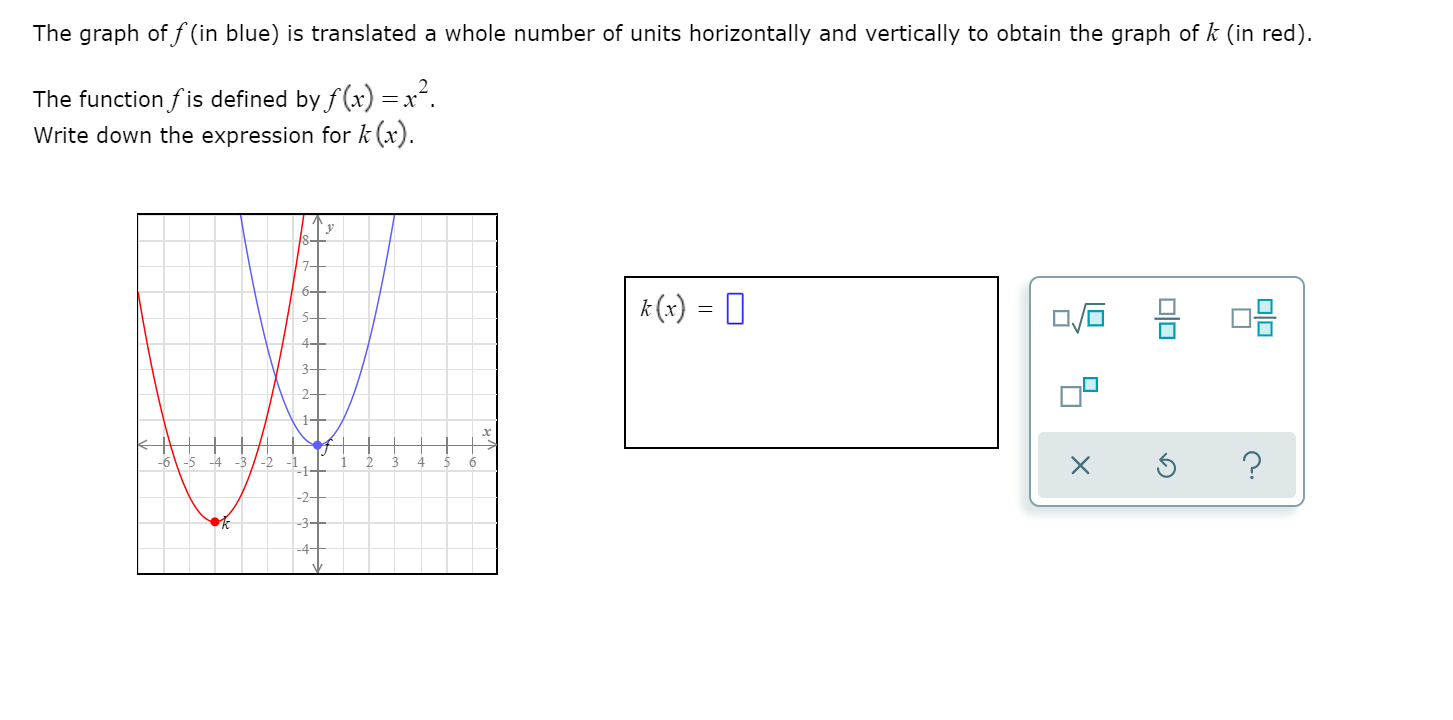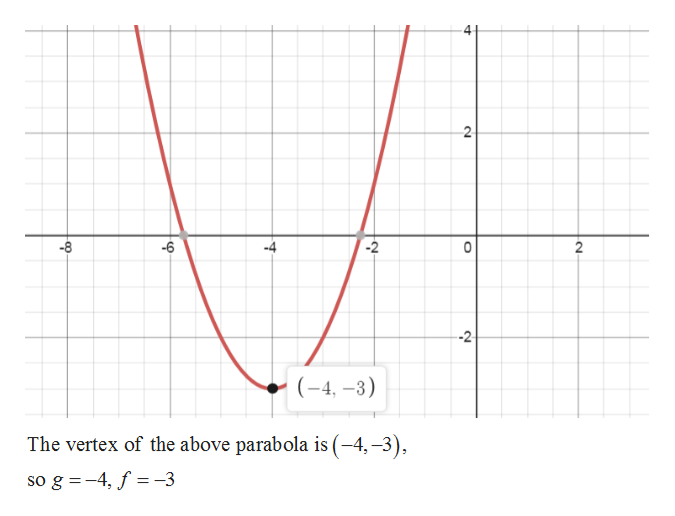# The graph of f (in blue) is translated a whole number of units horizontally and vertically to obtain the graph of k (in red).The function f is defined by f(x) =x.Write down the expression for k (x).6-k (x) = 05--1--2--3-olo

Question
194 views

Picture includedhelp_outlineImage TranscriptioncloseThe graph of f (in blue) is translated a whole number of units horizontally and vertically to obtain the graph of k (in red). The function f is defined by f(x) =x. Write down the expression for k (x). 6- k (x) = 0 5- -1- -2- -3- olo fullscreen
check_circle

Step 1

Given function and graph of function is

Step 2

The given graph is of the form of parabola. Suppose the general equation of the graph k(x) is

Step 3

The given graph of ...help_outlineImage Transcriptionclose-2 -8 -6 --2 (-4, –3) The vertex of the above parabola is (–4,–3), so g =-4, f = -3 4. 2. fullscreen

### Want to see the full answer?

See Solution

#### Want to see this answer and more?

Solutions are written by subject experts who are available 24/7. Questions are typically answered within 1 hour.*

See Solution
*Response times may vary by subject and question.
Tagged in

### Algebra